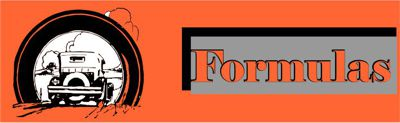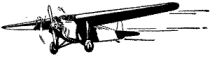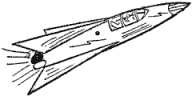Using the distance formula, it can be demonstrated how any one of the variable values can be found. Given that d = r t then r = d / t and t = d / t. Stated in words: If distance is the product of rate and time, then, to find either the rate or the time, simply divide the distance by the one known value.An airplane traveled 480 miles in 3 hours. What was its speed? 480 / 3 = 160 mphA rocket traveled at 1200 mph for a distance of 14400 miles. How long did it take to travel that distance? 14400 / 1200 = 12 hours
 The same plane went 600 miles in 4 hours. What was its speed? If the rocket traveled at a speed of 1600 mph for a distance of 5600 miles, how long would it have been traveling?